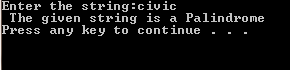# C++ Program TO Check For Palindrome String

/* A palindrome word is a word, whose appearance are the same in either forward or reverse direction. Examples: civic etc.In this program user will be prompted to enter the word & the program loop will test the condition of the string is Palindrome or not */

`#include <iostream>`
`#include <stdio.h>  ``//For gets() & system() functions`
`using` `namespace` `std;`
`int` `main()`
`{`
`    ``char` `s;`
`    ``int` `i,j,flag=0,len;`
`    ``cout<<``"Enter the string:"``;`
`    ``gets(s);`
`    ``len=``strlen``(strn);`
`    ``for``(i=0,j=len-1;i<=(len/2);++i,--j)`
`    ``{`
`    ``if``(s[i]==s[j])`
`    ``flag=1;`
`    ``}`
`    ``if``(flag)`
`    ``cout<<``"The given string is a Palindrome\n"``;`
`    ``else`
`    cout<<``"The given string is NOT a palindrome\n"``;`
system("pause");
return 0;
` ``}`

`Screenshot:`C++ Program TO Check For Palindrome String
` `` `